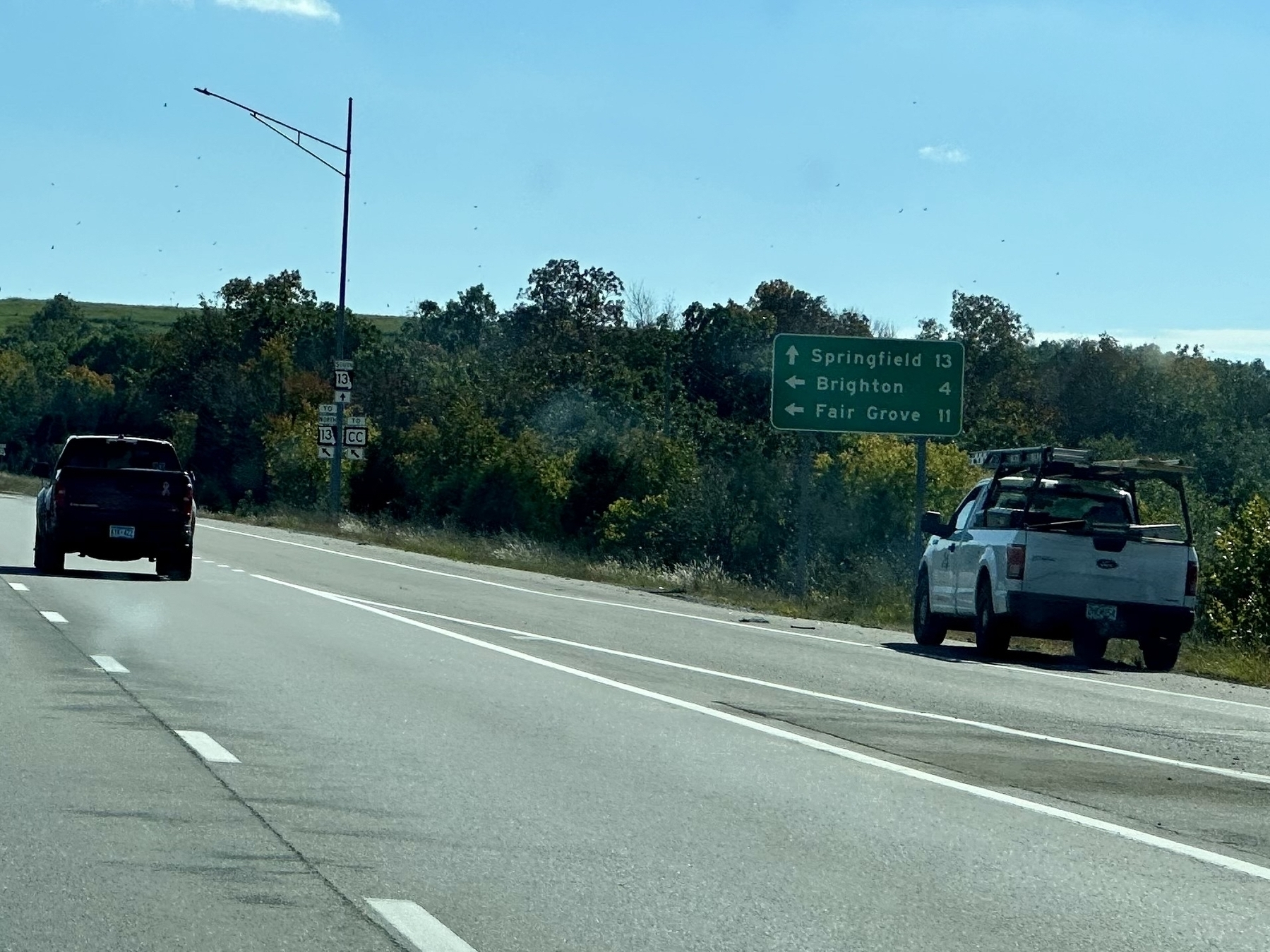$$\sqrt { 5 - 1 } = 2$$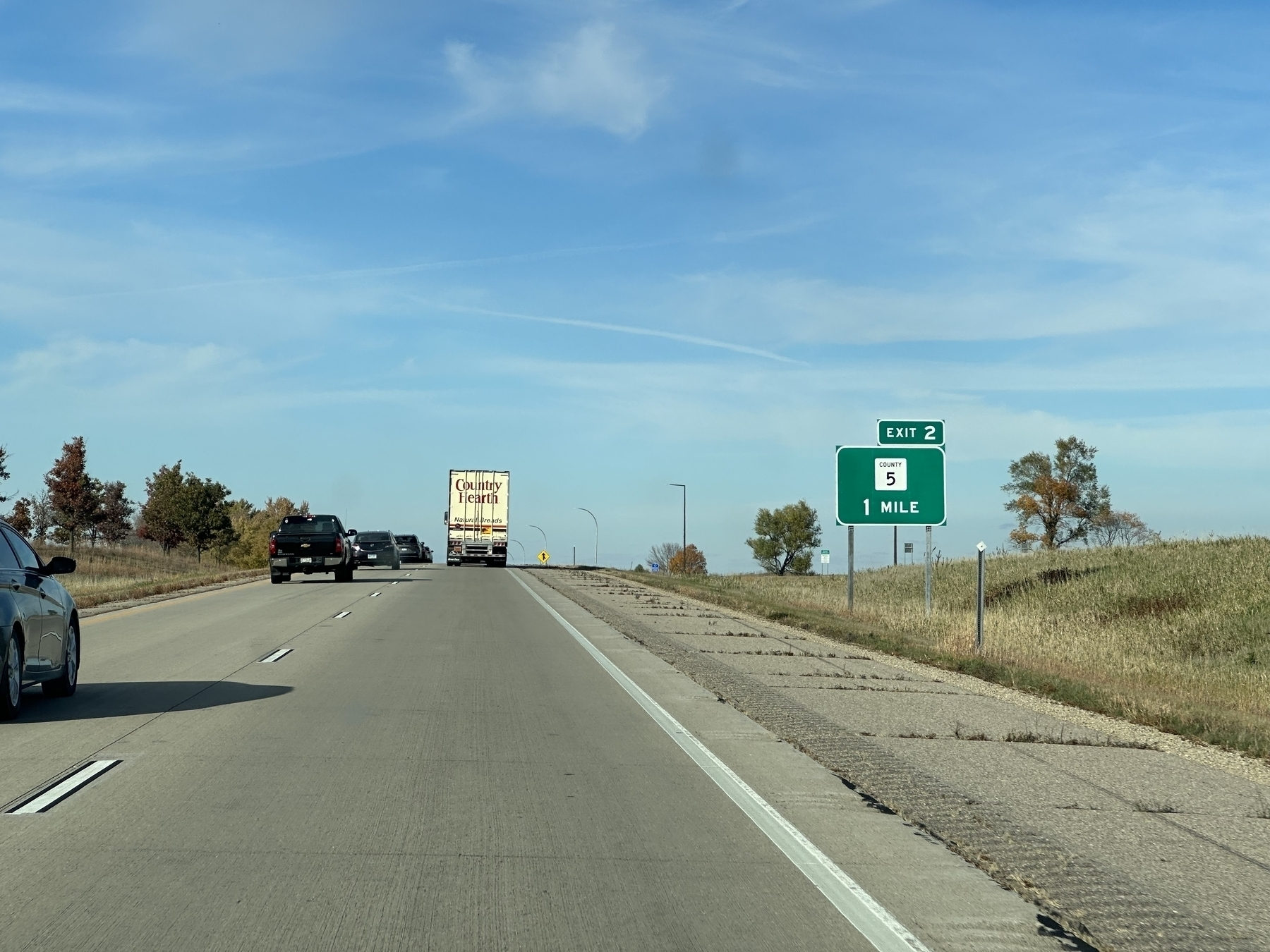## Mason Math

$$105 - 5 = 136 - 36$$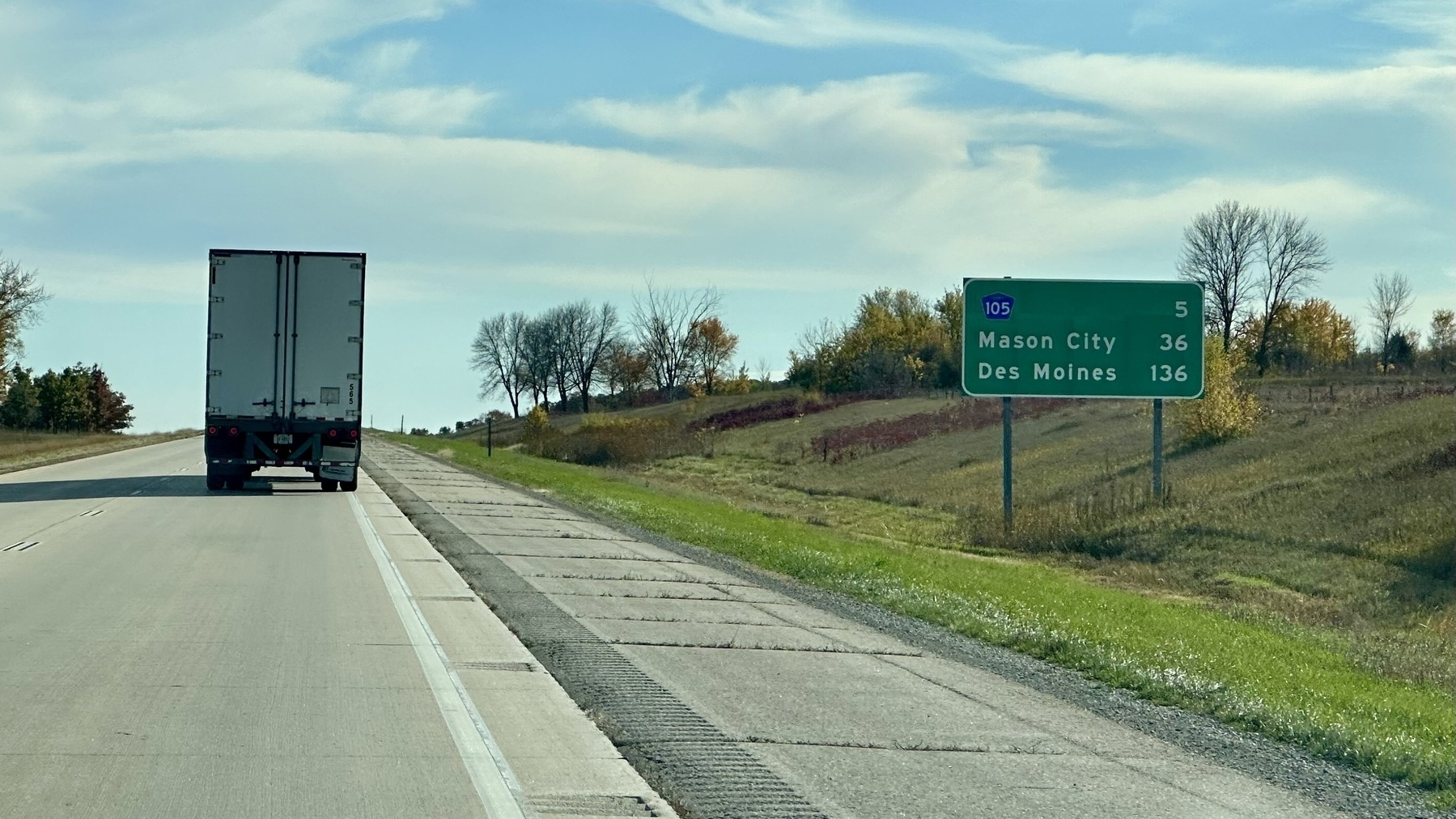## Iconium

$$7 + 12 = 19$$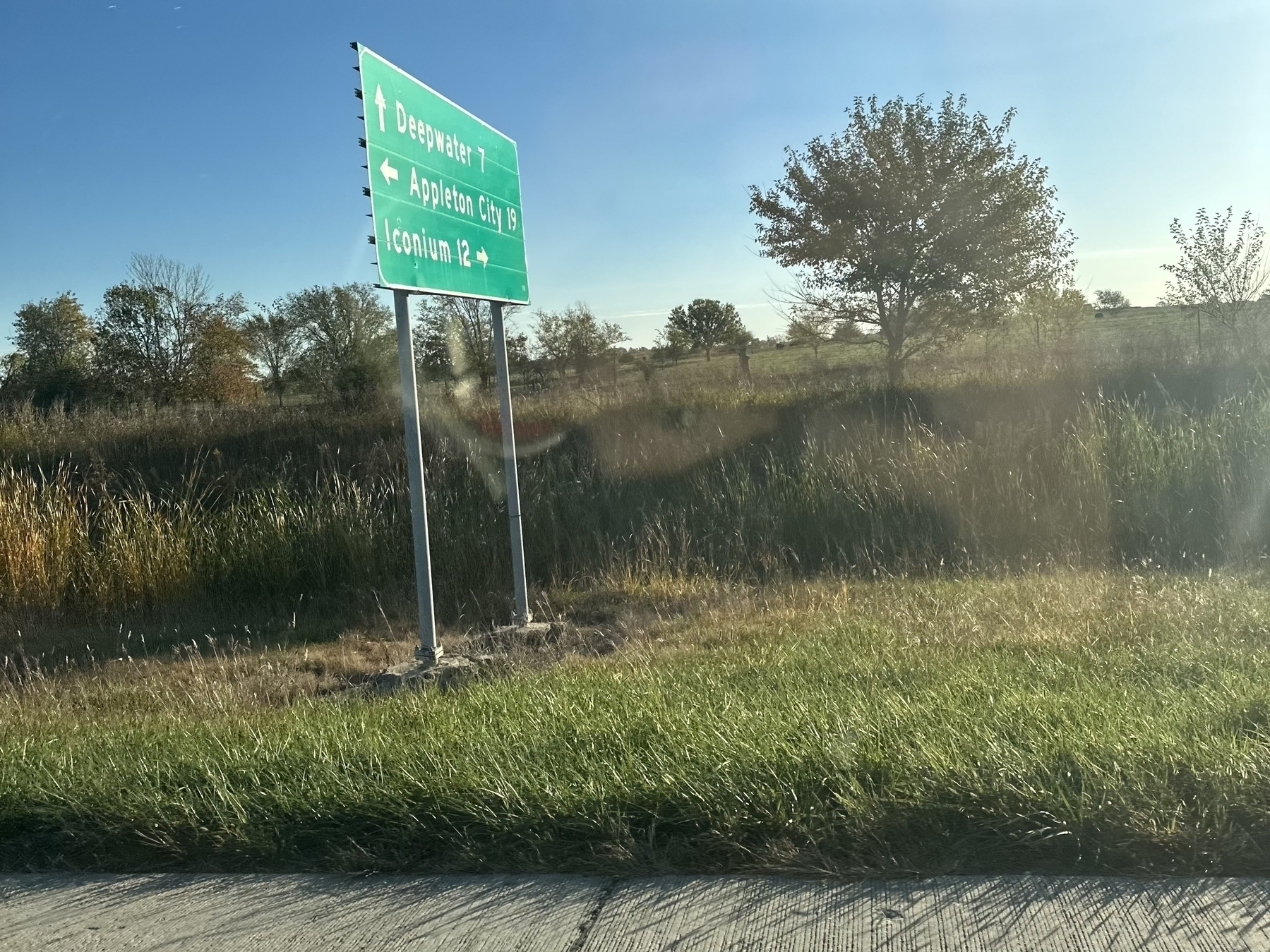$$5 + 1 = 6$$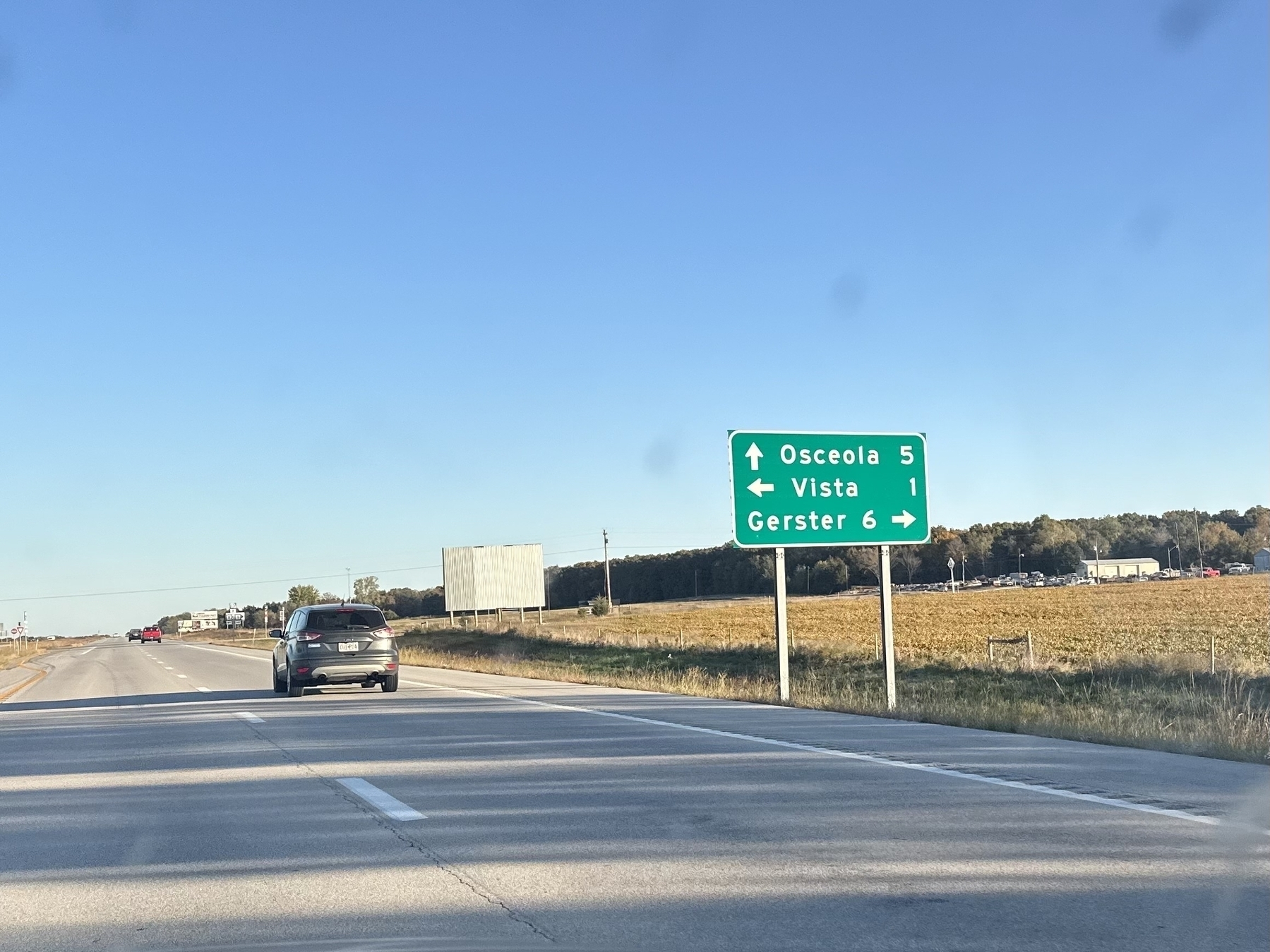$$10 + \sqrt 4 = 12$$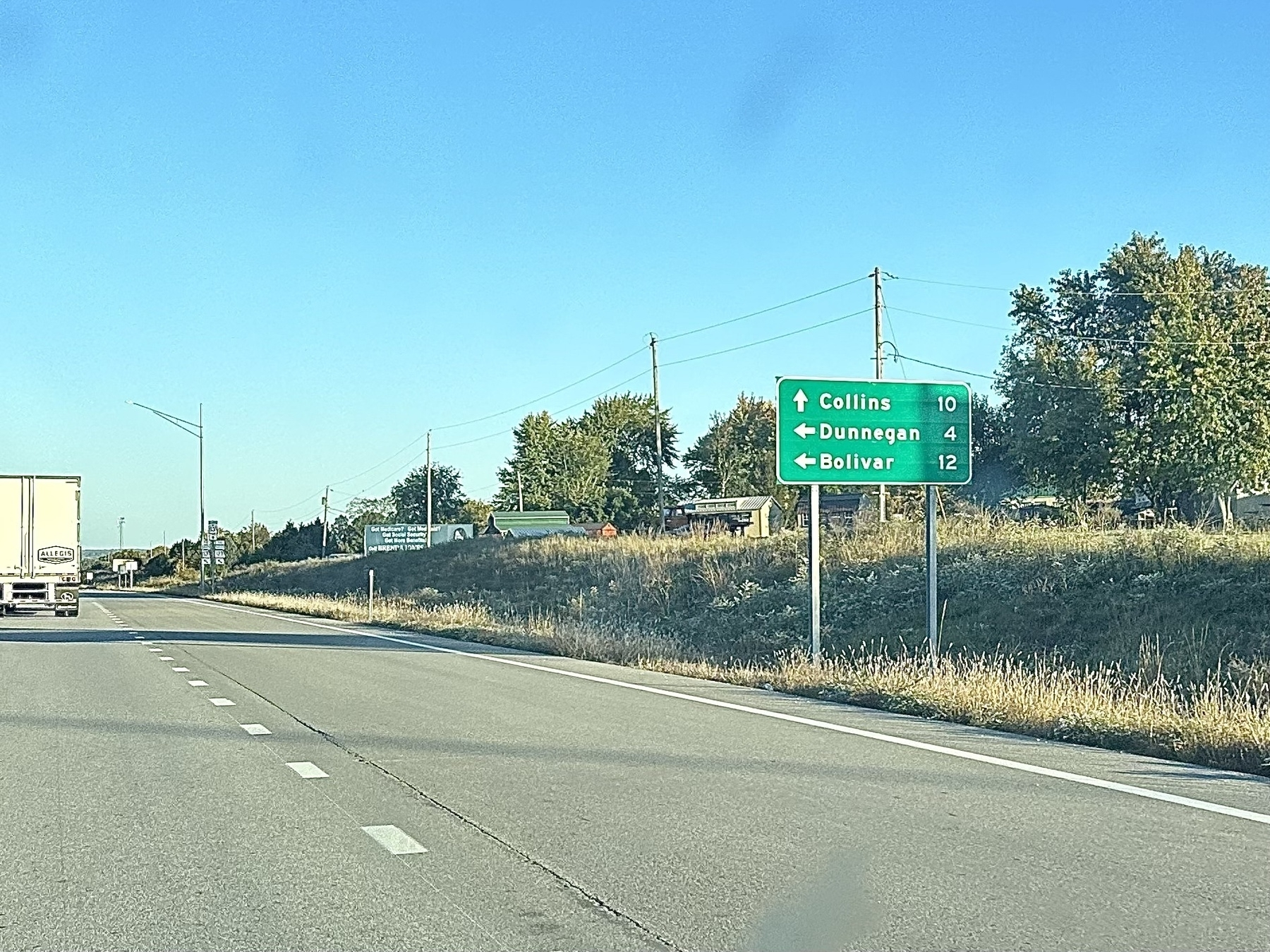## Genesis (2023)

All things have a beginning and the quest for mathematically significant road signs starts with this very sign.

$$3 \times 33 = 99$$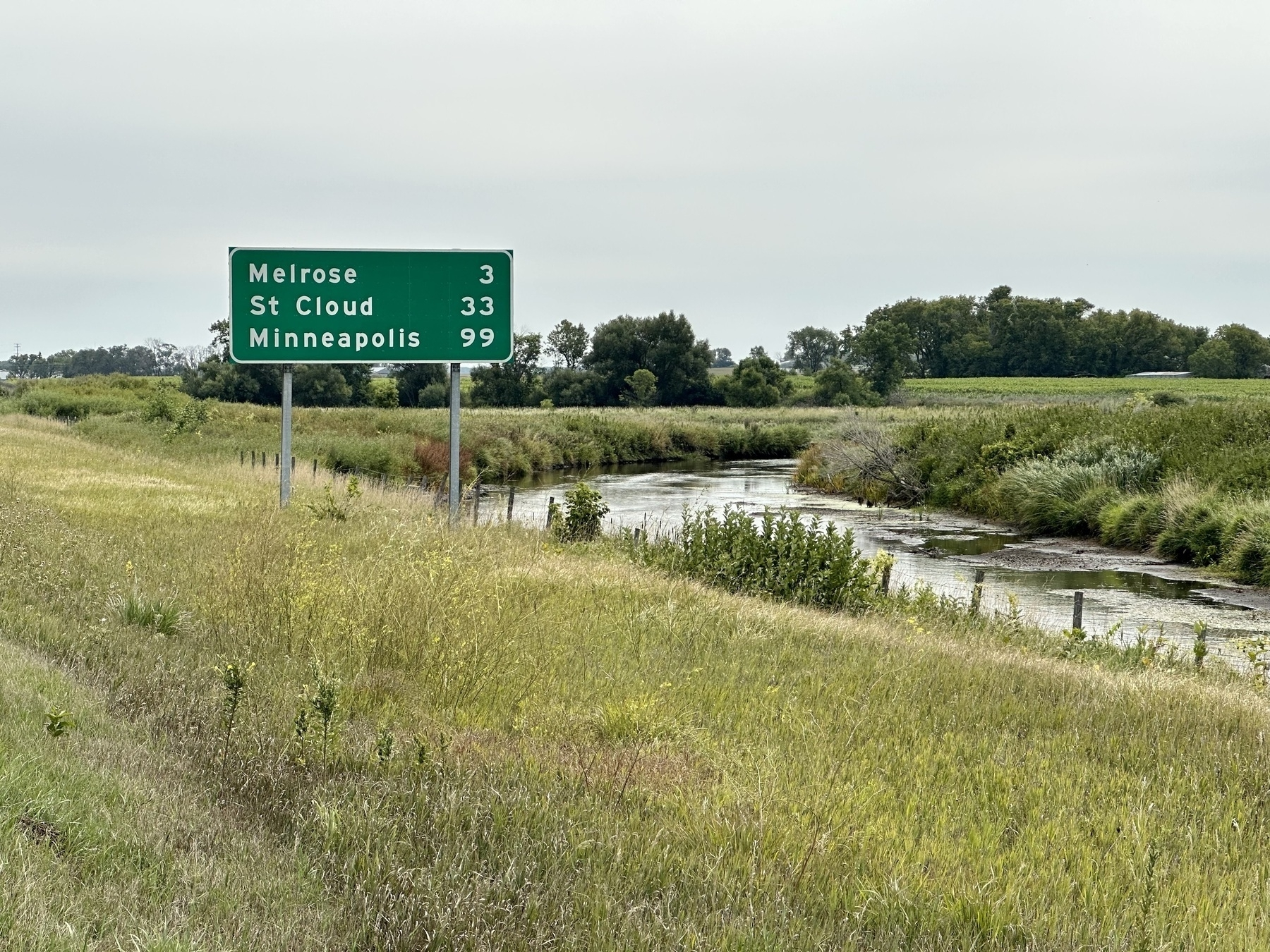This sign is on I-94 East as you drive toward the Twin Cities. Each time I would drive by it when returning from North Dakota I would see the math before I would see the cities on the sign.

“I wonder how many road signs have mathematical relationships like that?”

And thus Road Sign Math was born.

Given the importance of this sign, I pulled over and got a selfie with the sign itself.Also see Road Sign Math Rebooted!

## Fair Play

$$\sqrt { 83 - \sqrt 4 } = 6 + 3$$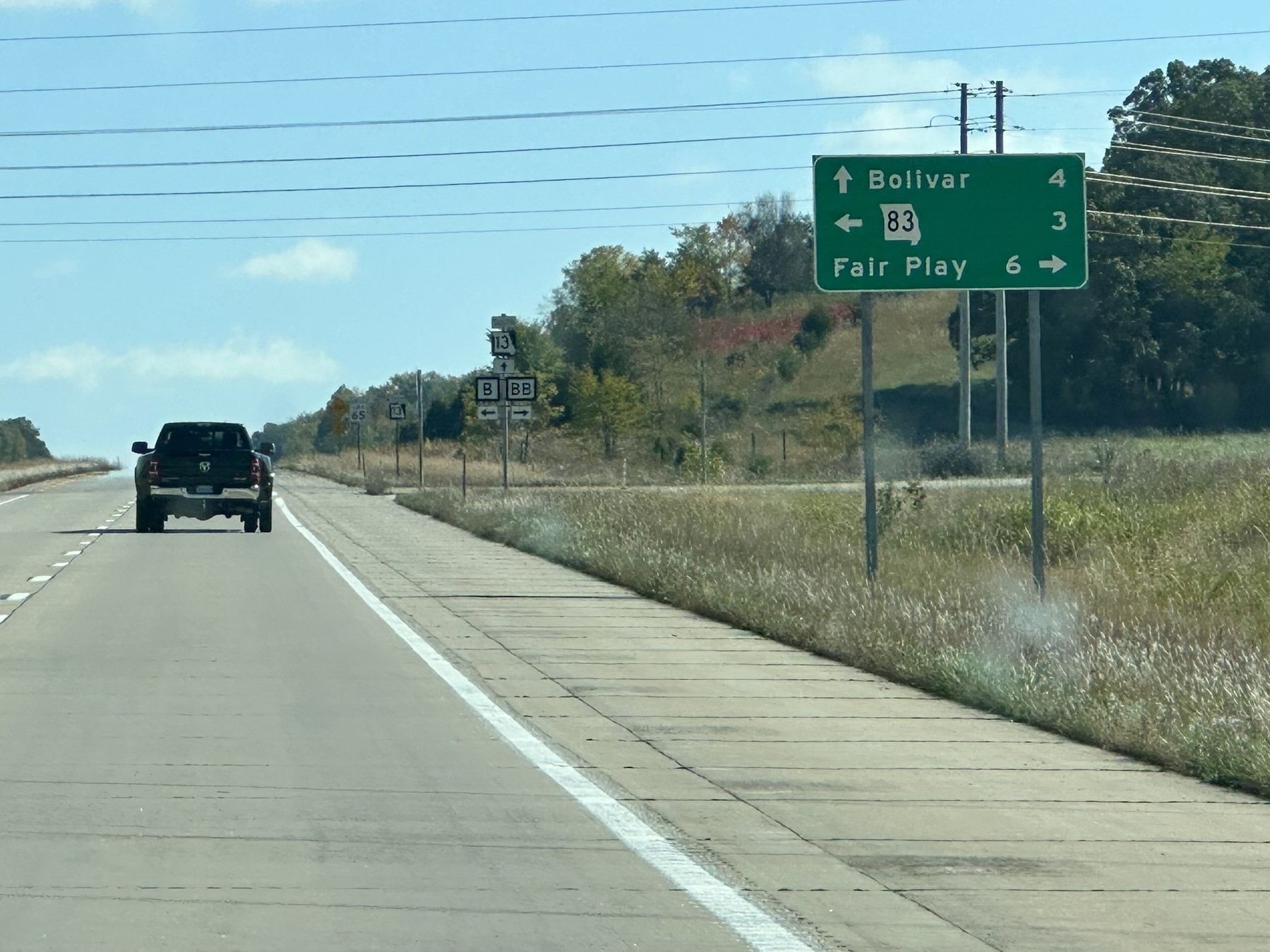## Fair Grove

$$11 + \sqrt 4 = 13$$